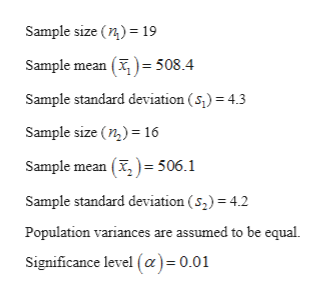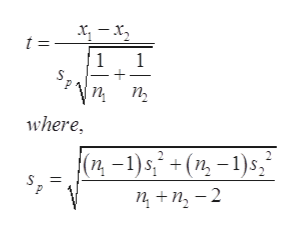# the concentration of heaxane 0a common solvent) was measured in units of micrograms per liter for a simple random sample of ninteen specimens of untreated ground water taken near a municipal landfill. the sample mean was 508.4 with a sample standard deviation of 4.3. sixteen specimens of treated ground water had an average hexane concentration of 506.1. with a standard deviation of 4.7. it is responsable to assume that both sample come from populations that are approximately normal. can you conclude that the mean hexane concentration is less in treated water than in untreated water? use the α= 0.01 level of significance.A). yes    B). No

Question
17 views

the concentration of heaxane 0a common solvent) was measured in units of micrograms per liter for a simple random sample of ninteen specimens of untreated ground water taken near a municipal landfill. the sample mean was 508.4 with a sample standard deviation of 4.3. sixteen specimens of treated ground water had an average hexane concentration of 506.1. with a standard deviation of 4.7. it is responsable to assume that both sample come from populations that are approximately normal. can you conclude that the mean hexane concentration is less in treated water than in untreated water? use the α= 0.01 level of significance.

A). yes    B). No

check_circle

Step 1

The provided information are:help_outlineImage TranscriptioncloseSample size (n)19 Sample mean () 508.4 Sample standard deviation (s) 4.3 Sample size (n2)16 Sample mean () 506.1 Sample standard deviation (s2) 4.2 Population variances are assumed to be equal Significance level (a)= 0.01 fullscreen
Step 2

The null and alternative hypotheses are:

Step 3

The test is left-sided.

The test stati...help_outlineImage Transcriptioncloset = 1 1 п, п, where (п -1)s + (п, -1)s, 2 nn-2 fullscreen

### Want to see the full answer?

See Solution

#### Want to see this answer and more?

Solutions are written by subject experts who are available 24/7. Questions are typically answered within 1 hour.*

See Solution
*Response times may vary by subject and question.
Tagged in

### Statistics